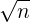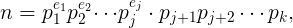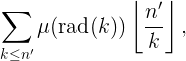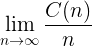# 题目

Prolblem 578: Integers with decreasing prime powers

Any positive integer can be written as a product of prime powers: p1a1 × p2a2 × ... × pkak,
where pi are distinct prime integers, ai > 0 and pi < pj if i < j.

A decreasing prime power positive integer is one for which ai ≥ aj if i < j.
For example, 1, 2, 15=3×5, 360=23×32×5 and 1000=23×53 are decreasing prime power integers.

Let C(n) be the count of decreasing prime power positive integers not exceeding n.
C(100) = 94 since all positive integers not exceeding 100 have decreasing prime powers except 18, 50, 54, 75, 90 and 98.
You are given C(106) = 922052.

Find C(1013).

# 暴力破解

`````` 1: using System;
2: using Skyiv.Utils;
3:
4: static class E578A
5: {
6:   static int[] primes;
7:
8:   static int C(int n, int i, int e)
9:   {
10:     int p = primes[i], z = 0;
11:     if (p > n) return 1;
12:     for (int j = 0; j <= e && n >= 1; ++j, n /= p)
13:       z += C(n, i + 1, (j != 0) ? j : e);
14:     return z;
15:   }
16:
17:   static void Main()
18:   {
19:     int n = (int)1e6;
20:     primes = Primes.GetPrimes(0, n, true);
21:     Console.WriteLine(C(n, 0, 64));
22:   }
23: }
``````

• 第 20 行的 Primes.GetPrimes 方法返回不超过`n`的所有素数，且尾部附加有一个哨兵，该哨兵的值大于`n`，但不一定是素数。该方法请参见参考资料。
• 第 8 至 15 行 C(n, i, e) 方法，就是通过递归调用自身来计算 C(n) 的。其中，`i`是各个素数的下标，`e`是各个素数的所允许的最大指数。
• 第 21 行给出的`e`的初值是 64，这是因为第一个素数是 2，而`n`不可能大于 264
• 第 11 行，如果素数`p`大于`n`，说明已经递归到底了，没有新的解，直接返回 1 （表示已经找到一个解）。
• 第 12 行，对指数`j`进行循环，从 0 开始，直到最大指数`e`。指数每增 1，`n`就要除以`p`。如果达到最大指数之前，`n`已经降到 1，则停止循环。
• 第 13 行，递归调用 C 方法自身，以完成计算。此时，往右移一个素数（`i + 1`）。如果当前指数`j`是 0，说明当前乘数是 1，即缺失当前位置的素数`p`，则指数限制为传入的指数`e`（即其左边的指数），否则指数限制为`j`，表示其右边的指数不能超过当前位置的指数。

• 首先，n = 1013 已经超过 int.MaxValue = 231 - 1，这个程序需要使用不超过 n 的所有素数，我们的计算机不可能有这么多的内存。
• 其次，这个程序是一个个累加计算的，对于很大的数，递归调用会导致堆栈溢出。
• 最后，对于很大的数，即使有无限的堆栈，运行时间也太长了。

# 改进

`````` 1: using System;
2: using Skyiv.Utils;
3:
4: static class E578
5: {
6:   static int[] primes;
7:   static PrimeHelper helper;
8:
9:   static long C(long n, int i, int e)
10:   {
11:     long p = primes[i], z = 0;
12:     if (p * p > n) z = Math.Max(0, helper.π(n) - i) + 1;
13:     else for (int j = 0; j <= e && n >= 1; ++j, n /= p)
14:       z += C(n, i + 1, (j != 0) ? j : e);
15:     return z;
16:   }
17:
18:   static void Main()
19:   {
20:     long n = (long)1e13;
21:     Console.WriteLine("n = {0:N0}", n);
22:     helper = new PrimeHelper(n);
23:     primes = helper.GetPrimes(true);
24:     Console.WriteLine("π(√n) = {0:N0}", primes.Length-1);
25:     Console.WriteLine("C(n) = {0:N0}", C(n, 0, 64));
26:   }
27: }
``````

• 第 22 行的 PrimeHelper 类请见参考资料。
• 第 23 行得到不超过的所有素数，尾部附加有一个哨兵（该哨兵的值大于，但不一定是素数）。注意，这里只要求不超过的素数，而上一小节的程序要求不超过 n 的素数。
• 第 9 至 16 行的 C 方法基本上与上一小节的程序相同，除了第 12 行。
• 第 12 行，当 p2 > n 时，只能乘以一个素数（的 1 次方）。所以返回 π(n) - π(p-1) + 1。
• 此时，π(p-1) == i（注意，i 的初值是 0，而不是 1）。
• 还要注意边界情况，当 p > n 时，π(n) - π(p-1) 有可能是负数，需要调整为 0。
• 加 1 是因为除了素数以外，乘以 1 也算一个。

# 运行过程分析

n = 71
π(√n) = 4
C(n) = 68• 19 = 17+1+1, 17 = π(71)-4+1, 1 = π(4)-4+1, 1 = Max(0,π(1)-4)+1
• 24 = 19+4+1, 4 = π(14)-3+1, 1 = Max(0,π(2)-3)+1
• 36 = 24+8+3+1, 8 = π(23)-2+1, 3 = π(7)-2+1, 1 = Max(0,π(2)-2)+1
• 11 = 9+2, 9 = π(35)-3+1, 2 = π(7)-3+1
• 15 = 11+4, 4 = π(11)-2+1
• 9 = 6+2+1, 6 = π(17)-2+1, 2 = π(5)-2+1, 1 = Max(0,π(1)-2)+1
• 68 = 36+15+9+4+2+1+1, 4 = π(8)-1+1, 2 = π(4)-1+1, 1 = π(2)-1+1, 1 = Max(0,π(1)-1)+1

# 更快的程序

I wrote n aswhere ei > 1. The left part (with powers) is easy to enumerate with brute force, and the right part can be counted aswhere the sum is over all numbers with prime factors less than pj+1 in the first power, and others in the second. 26 seconds in OCaml.# 附加题

1. 请问以下极限是否存在：2. 如果存在，它是代数数还是超越数？